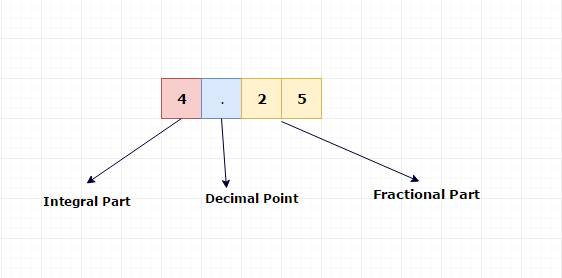# How to convert floating numbers into binary numbers ?

Let’s take an example

#### 4.25

Where,

4 is an integral part.

0.25 is a fractional part.

#### Pictorial Explanation#### Integral Part (4)

To convert an integral part into binary, just follow the previously discussed method.

Binary Number System

Using that method, we can represent 4 as (100) 2.

#### Fractional part (0.25)

To convert the fractional part to binary, multiply fractional part with 2 and take the one bit which appears before the decimal point.

Follow the same procedure with after the decimal point (.) part until it becomes 1.0.

Like,

0.25 * 2 =0.50 //take 0 and move 0.50 to next step

0.50 * 2 =1.00 //take 1 and stop the process

0.25 = (01) 2

Combining both integral and fractional,

4.25 = (100.01) 2

## Example

10.75

10 = (1010) 2

#### Fractional Part

0.75 * 2 =>1.50 // take 1 and move .50 to next step

0.50 * 2 =>1.00 // take 1 and stop the process because no remainder

0.75 = (11) 2

Combining both integral and fractional,

10.75 = (1010.11) 2

## Example

2.33

2 = (10) 2

#### Fractional Part

0.33 * 2 =>0.66 // take 0 and move .66 to next step

0.66 * 2 =>1.32 // take 1 and move .32 to next step

0.32 * 2 =>0.64 // take 0 and move .64 to next step

0.64 * 2 =>1.28 // take 1 and move .28 to next step

0.28 * 2 =>0.56 // take 0 and move .56 to next step

0.56 * 2 =>1.12 // take 1 and move .12 to next step

0.12 * 2 =>0.24 // take 0 and move .24 to next step

0.24 * 2 =>0.48 // take 0 and move .48 to next step

0.48 * 2 =>0.96 // take 0 and move .96 to next step

0.96 * 2 =>1.92 // take 1 and move .92 to next step

0.92 * 2 =>1.84 // take 1 and move .84 to next step

0.84 * 2 =>1.68 // take 1 and move .68 to next step

0.68 * 2 =>1.36 // take 0 and move .36 to next step

0.36 * 2 =>0.72 // take 0 and move .72 to next step

0.72 * 2 =>1.44 // take 1 and move .44 to next step

0.44 * 2 =>0.88 // take 0 and move .88 to next step

0.88 * 2 =>1.76 // take 1 and move .76 to next step

0.76 * 2 =>1.32 // take 1 and move .32 to next step

Here, the fractional part 0.32 which is repeating again.

And Some fractional part numbers will not end up with 1.0 with the above method.

In floating number storage, the computer will allocate 23 bits for the fractional part. So, it's enough to do the above method at max 23 times.

2.33 = (10.010101000111001011) 2